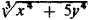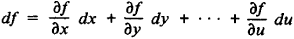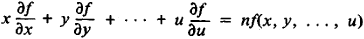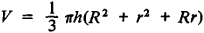# Homogeneous Function

(redirected from Euler's homogeneous function theorem)

## homogeneous function

[‚hä·mə′jē·nē·əs ′fəŋk·shən]
(mathematics)
A real function ƒ(x1, x2, …, xn ) is homogeneous of degree r if ƒ(ax1, ax2, …, axn ) = a rƒ(x1, x2, …, xn ) for every real number a.

## Homogeneous Function

a function of one or several variables that satisfies the following condition: when all independent variables of a function are simultaneously multiplied by the same (arbitrary) factor, the value of the function is multiplied by some power of this factor. In algebraic terms, a function f(x, y, …, u) is said to be homogeneous of degree n if for all values of x, y, …, u and for any λ

fx, λy, …, λu) = λnf(x, y, …, u)

For example, the functions x2 – 2y2, (xy – 3z)/(z2 + xy), andare homogeneous of degree 2, –1, and 4/3, respectively. Functions homogeneous of degree n are characterized by Euler’s theorem that asserts that if the differential of each independent variable is replaced with the variable itself in the expression for the complete differentialthen we obtain the function f(x, y, …, u) multiplied by the degree of homogeneity:Homogeneous functions are frequently encountered in geometric formulas. In the equation x = f(a, b, …, l), where a, b, …, l are the lengths of segments expressed in terms of the same unit, f must be a homogeneous function (of degree 1, 2, or 3, depending on whether x signifies length, area, or volume). For example, in the formula for the volume of a truncated coneV is a homogeneous function of degree 3 in h, R, and r.

Site: Follow: Share:
Open / Close